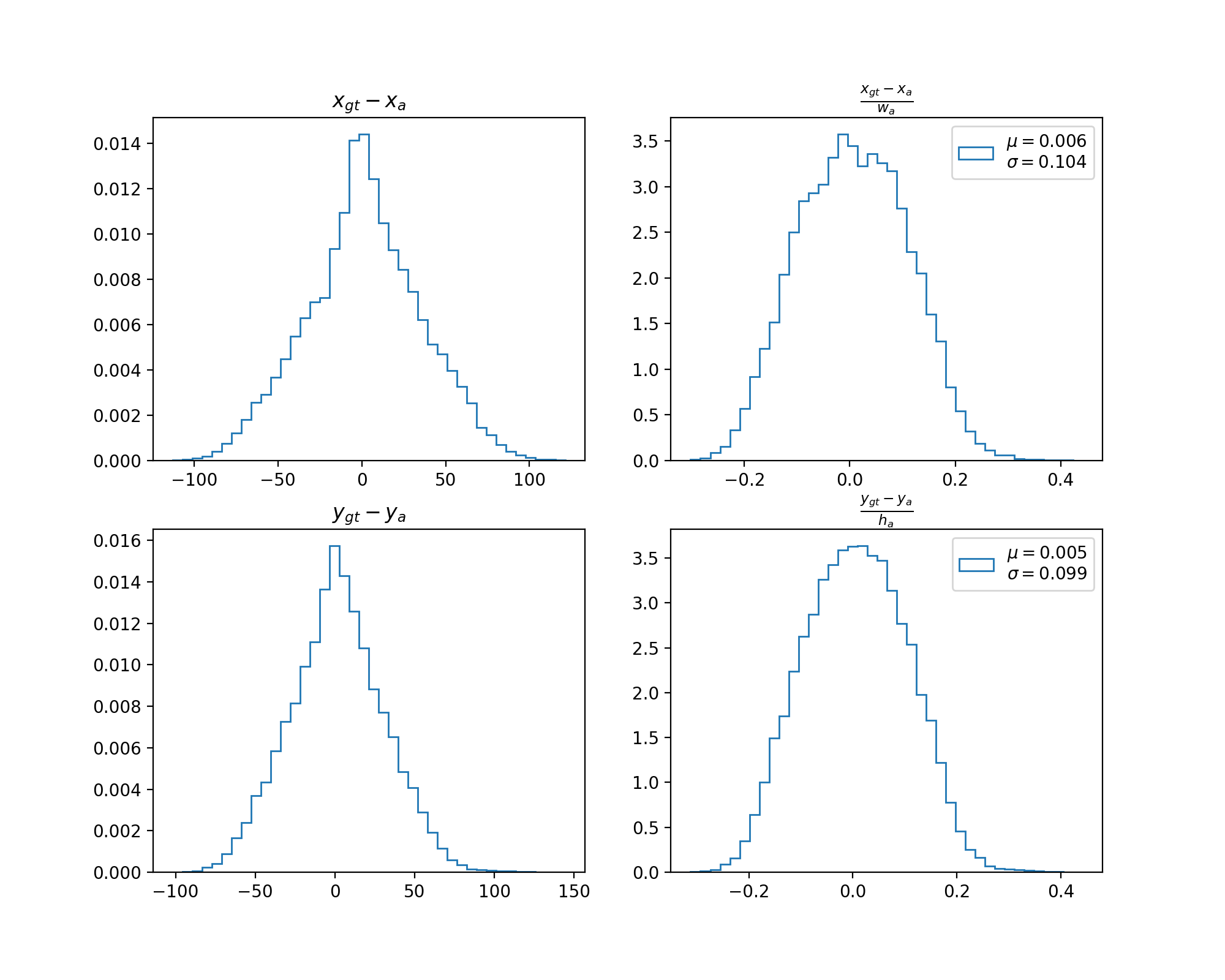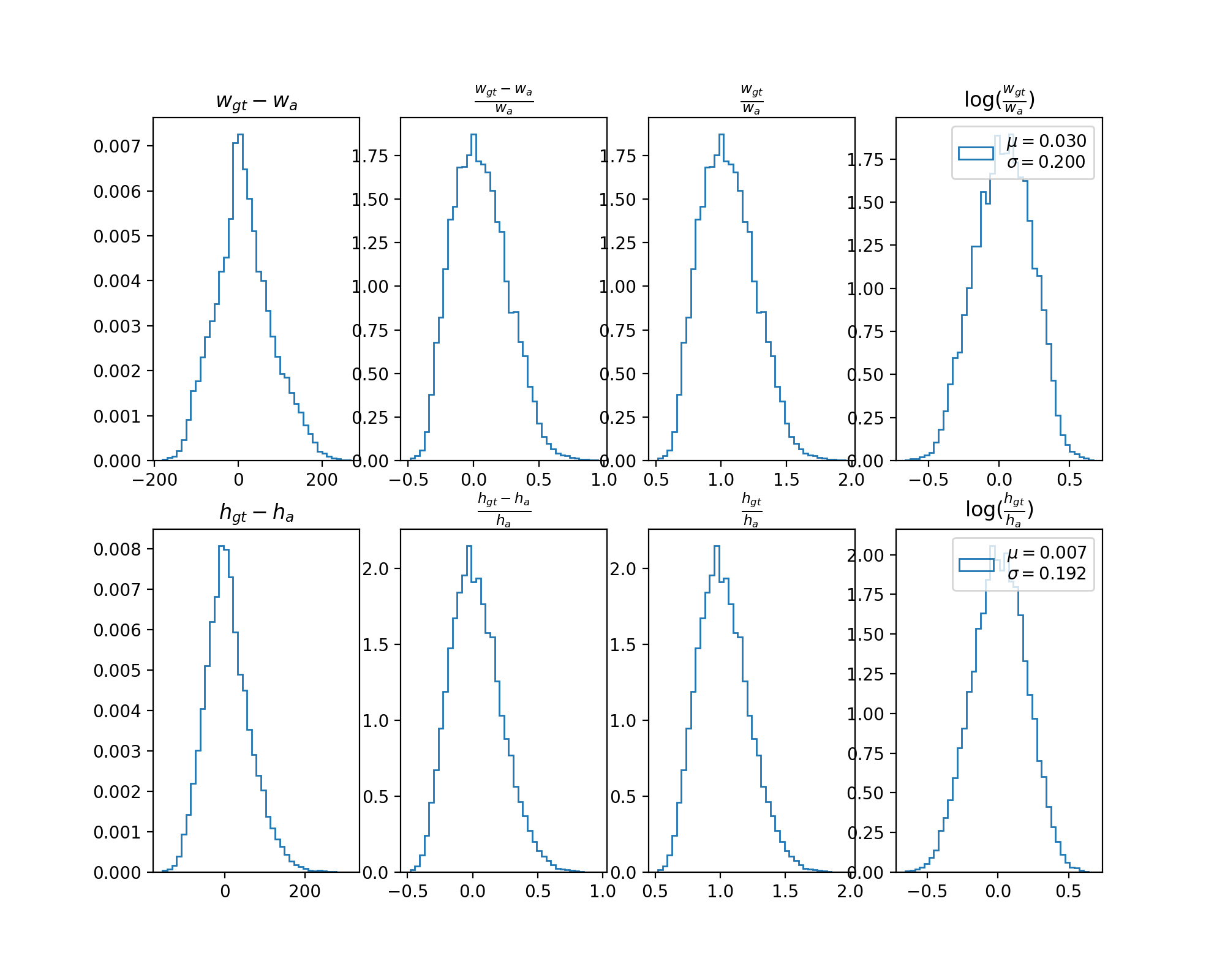# 目标检测中真实框与其匹配的锚框之间的关系1) $x_{gt} - x_a$ 和 $\frac{x_{gt} - x_a}{w_a}$ 的直方图接近均值为 0 的正态分布.

2) $w_{gt} - w_a$ 和 $\log\frac{w_{gt}}{w_a}$ 的直方图接近均值为 0 的正态分布.

3) $\frac{w_{gt} - w_a}{w_a}$ 和 $\frac{w_{gt}}{w_a}$ 的直方图左右明显不对称.

1) 使用 $\frac{x_{gt} - x_a}{w_a}$ 而不是 $x_{gt} - x_a$ 来对 $x_{gt}$ 进行编码的原因是前者具有尺度不变性.

2) $\frac{w_{gt} - w_a}{w_a}$ 和 $\frac{w_{gt}}{w_a}$ 的直方图左右明显不对称, 与正态分布不相符, 所以均不选来对 $w_{gt}$ 进行编码.

3) 使用 $\log\frac{w_{gt}}{w_a}$ 而不是 $w_{gt} - w_a$ 来对 $w_{gt}$ 进行编码的原因是前者具有尺度不变性.

4) $\frac{x_{gt} - x_a}{x_a}$ 和 $\log\frac{w_{gt}}{w_a}$ 的分布的标准差并不相同, 而一般的定位 loss 是无差别的优化中心坐标和宽高的, 所以最好将两者的分布变成一样. 由此可以解释一些代码实现中为何要对编码后的中心和宽高除以一个数 (中心坐标和宽高一般对应不同的值). Bounding-Box-Encoding-Decoding 提到对编码后的中心和宽高进行归一化, 即除以统计的标准差, 个人认为要不要进行归一化与定位 loss 的类型有关 (个人观点如果是 L1 和 L2 Loss, 无需进行归一化, 如果是 Smooth L1 Loss, 最好进行归一化).

• 20211014, 发布